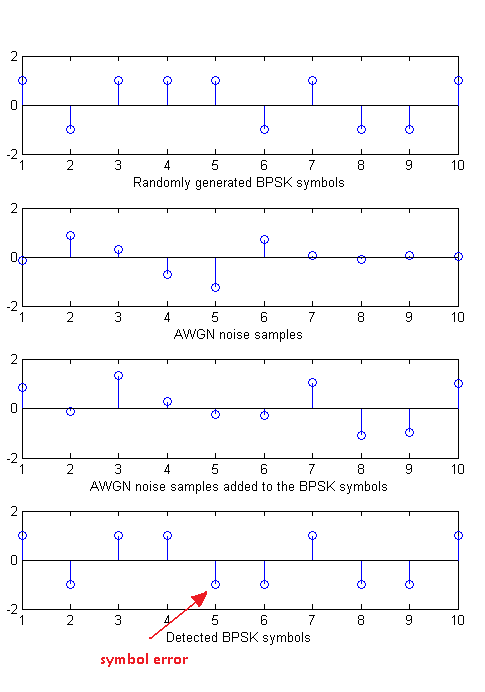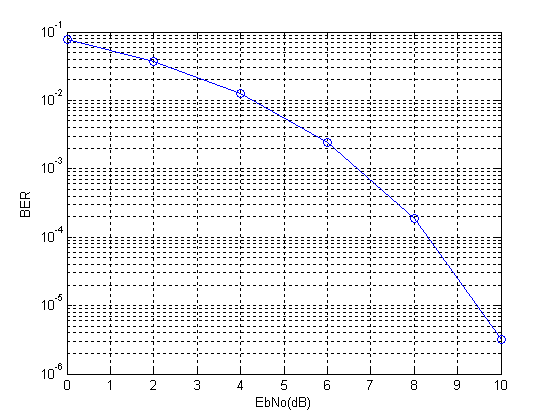# Shannon Capacity of a GSM Channel

We know that GSM bit rates can vary from a few kbps to a theoretical maximum of 171.2kbps (GPRS). But what is the actual capacity of a 200kHz GSM channel. We can use the Shannon Capacity Theorem to find this capacity.

C=B*log2(1+SNR)

or

C=B*log2(1+P/N)

The noise power can be found by using the following formula:

N=B*No=k*T*B=(1.38e-23)*(293)*(200e3)=8.08e-16W=-121dBm

Let us now assume a signal power 0f -90dBm. This gives us an SNR of 31dB or 1258.9 on linear scale. The capacity can thus be calculated as:

C=200e3*log2(1+1258.9)=2.06Mbps

This is the capacity if all time slots are allocated to a single user. If only one time slot is allocated to a user the capacity would be reduced to 257.48kbps.

# WCDMA Capacity (Mbps)

The capacity of any wireless communication channel is given by the well known Shannon Capacity Theorem:

C=B*log2(1+SNR)

or

C=B*log2(1+P/(NoB))

where C is the capacity of the channel in bits/sec, P in the noise power in Watts, No is the noise power spectral density in Watts/Hz and B is the channel bandwidth in Hz. It is obvious that the channel capacity increases with increase in signal power. However, the relationship with bandwidth is a bit complicated. The increase in bandwidth decreases the SNR (keeping the signal power and noise power spectral density same). Therefore the capacity does not increase linearly with bandwidth.

It is assumed that the signal power is -80dBm and the noise power spectral density is -170dBm/Hz.

Let us now consider the case of a WCDMA system where the noise component consists of the AWGN noise as well Multiple Access Interference (MAI). The above capacity formula is then modified as:

C=B*log2(1+SINR)

or

C=B*log2(1+P/(NoB+(U-1)*P))

where U is the number of users.

It is assumed that WCDMA system has a bandwidth of 5MHz, signal power of -80dBm and noise power spectral density of -170dBm/Hz. It is observed that the capacity of the WCDMA system decreases exponentially with increase in number of users (all users are assumed to have equal power at the receiver). The capacity of a 5MHz channel drops from about 38Mbps for a single user to about 370kbps for 20 users (the combined capacity of 20 users is 7.4Mbps which is much lesser than the capacity of a single user in AWGN only).

Note:

1. In some references the signal power is adjusted by the processing gain but I think that this is not correct for capacity calculations because in that case the bandwidth should be adjusted as well.

2. The capacity in fading environments would be less than AWGN capacity. However, multi-antenna systems allow for higher capacities.

# Bit Error Rate of BPSK

Modulation is the process by which a binary stream (zeros and ones) is converted to a format that is suitable for transmission over a wired or wireless channel that is prone to noise and interference as well as distortion. The most basic modulation scheme is BPSK or Binary Phase Shift Keying. It transmits the information in the phase of the signal which could be one of two values (0 degrees or 180 degrees).

BPSK signal can be represented as (called the passband representation)

s(t)=a(t)*cos(2*pi*f*t)

where a(t) is a time varying parameter which can have one of two values (+1 or -1). This is equivalent to having the phase of the carrier rotated by 0 degrees or 180 degrees. In simulation of digital communications systems we usually take out the carrier and perform the simulation at baseband. The passband and baseband simulations are equivalent because the carrier signal introduced at the transmitter can be easily removed at the receiver by a process called correlation (or simply put multiplication by the carrier followed by low pass filtering) and what we are left with is the parameter a(t).

If the transmitted signal is given as

a(t)*cos(2*pi*f*t)

then by multiplication with the carrier at the receiver we get

a(t)*cos(2*pi*f*t)*cos(2*pi*f*t)

=(a(t)/2)*(1+cos(4*pi*f*t))

and after low pass filtering the cosine term at twice the carrier frequency is removed and we get the parameter a(t) scaled by the factor 1/2. Since the information is contained in the sign of the parameter a(t) we can recover our transmitted symbols.

So in simulation, instead of multiplying the parameter a(t) by the carrier at transmitter and then again at the receiver we simply transmit a(t). This is equivalent to simulation of a Pulse Amplitude Modulation (PAM) system with two levels. Following are the steps involved in the simulation of BPSK system.

Steps:

1. Generate a random sequence of symbols (+1,-1)

2. Generate samples of Additive White Gaussian Noise (AWGN) with the required variance (noise power = noise variance OR noise power = square of noise standard deviation OR noise power = noise power spectral density * signal bandwidth).

3. Add AWGN samples to the BPSK signal.

4. Detection is performed at the receiver by determining the sign of the parameter a(t).

5. And finally the bit error rate (BER) is calculated. Which is the same as symbol error rate (SER) in this case.

Given below is the MATLAB code that performs these functions. Also shown below are the signals generated at the first four steps and the bit error rate calculated in the fifth and last step.

```%%%%%%%%%%%%%%%%%%%%%%%%%%%%%%%%%%%%%%%%%%%%%%%%%%%%%%%%%%%%%%%%%%%%%
% FUNCTION TO CALCULATE BER OF BPSK IN AWGN
% l - Input, length of the symbol sequence
% EbNo - Input, energy per bit to noise power spectral density in dB
% ber - Output, bit error rate
%%%%%%%%%%%%%%%%%%%%%%%%%%%%%%%%%%%%%%%%%%%%%%%%%%%%%%%%%%%%%%%%%%%%%
function[ber]=err_rate(l,EbNo)
s=2*(round(rand(1,l))-0.5);             % Generate BPSK symbols
n=(1/sqrt(2*10^(EbNo/10)))*randn(1,l);  % Generate AWGN noise
r=s+n;                                  % Add noise to signal
s_=sign(r);                             % Detect symbols
ber=(l-sum(s==s_))/l;                   % Calculate BER
return
%%%%%%%%%%%%%%%%%%%%%%%%%%%%%%%%%%%%%%%%%%%%%%%%%%%%%%%%%%%%%%%%%%%%%
```

The length of the symbol sequence and EbNo (a bit different than SNR) are the inputs to the function and the bit error rate (BER) is the output. The length of the sequence must be such that you can count about 25 symbol errors at each value of EbNo. This means that at an EbNo of 10dB you would need to pass a few million symbols through the channel. Try it out!!!Note:

1. To generate the above given bit error rate plot you would have to create a piece of code which calls the above function for each value of EbNo and stores the output BER value in an array and then plot the BER vs EbNo at the end of simulation. We leave this to  you as an exercise.

2. We have generated BPSK symbols directly instead of first generating a binary sequence. This does not matter much in this simple example but for more advanced modulation schemes we would have to first generate a binary stream and then from that the symbols.

3. We have used one sample per symbol of BPSK modulation, as shown in the figure above. But sometimes we have to select higher number of samples per symbol (usually 4 to 10) to implement some other signal processing functions.

4. Most of the concepts discussed above can be extended to other digital modulation schemes. The concepts for analog modulation schemes are somewhat different and we do not use error rates to evaluate the performance of these schemes.